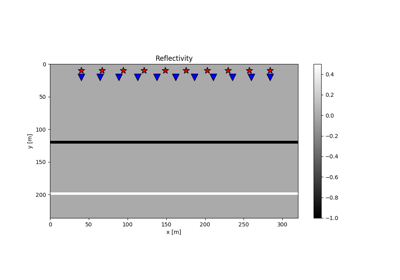# pylops.waveeqprocessing.LSM¶

class pylops.waveeqprocessing.LSM(z, x, t, srcs, recs, vel, wav, wavcenter, y=None, mode='eikonal', dottest=False)[source]

Least-squares Migration (LSM).

Solve seismic migration as inverse problem given smooth velocity model vel and an acquisition setup identified by sources (src) and receivers (recs)

Parameters: z : numpy.ndarray Depth axis x : numpy.ndarray Spatial axis t : numpy.ndarray Time axis for data srcs : numpy.ndarray Sources in array of size $$\lbrack 2/3 \times n_s \rbrack$$ recs : numpy.ndarray Receivers in array of size $$\lbrack 2/3 \times n_r \rbrack$$ vel : Velocity model of size $$\lbrack (n_y \times) n_x \times n_z \rbrack$$ (or constant) wav : numpy.ndarray Wavelet wavcenter : int Index of wavelet center y : numpy.ndarray Additional spatial axis (for 3-dimensional problems) mode : numpy.ndarray, optional Computation mode (eikonal, analytic - only for constant velocity) dottest : bool, optional Apply dot-test

Notes

Inverting a demigration operator is generally referred in the literature as least-squares migration (LSM) as historically a least-squares cost function has been used for this purpose. In practice any other cost function could be used, for examples if solver='pylops.optimization.sparsity.FISTA' a sparse representation of reflectivity is produced as result of the inversion.

Finally, it is worth noting that in the first iteration of an iterative scheme aimed at inverting the demigration operator, a projection of the recorded data in the model domain is performed and an approximate (band-limited) image of the subsurface is created. This process is referred to in the literature as migration.

Attributes: Demop : pylops.LinearOperator Demigration operator

Methods

 __init__(self, z, x, t, srcs, recs, vel, …) Initialize self. solve(self, d[, solver]) Solve least-squares migration equations with chosen solver
solve(self, d, solver=<function lsqr at 0x7fc33cc3bc80>, **kwargs_solver)[source]

Solve least-squares migration equations with chosen solver

Parameters: d : numpy.ndarray Input data of size $$\lbrack n_s \times n_r \times n_t \rbrack$$ solver : func, optional Solver to be used for inversion **kwargs_solver Arbitrary keyword arguments for chosen solver minv : np.ndarray Inverted reflectivity model of size $$\lbrack (n_y \times) n_x \times n_z \rbrack$$

## Examples using pylops.waveeqprocessing.LSM¶13. Least-squares migration